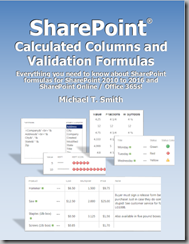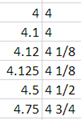## 3/26/2018

### Adding Fractions (1/4) to SharePoint Calculated Columns

(The following works SharePoint 2010, 2013, 2016, SharePoint Online, and most likely, 2019!)

0, 1/8, 1/4, 3/8, 1/2, 5/8, 3/4, and 7/8

This is a sample from my soon to be published book “SharePoint Calculated Columns and Validation Formulas”. If you would like to see a lot more about what you can do with Calculated Columns, and maybe win a free book, attend my session at SharePoint Cincy 2018 on April 10th!In Excel you can get fractions using =TEXT(yourNumberColumn,"# ?/?"):The same function in SharePoint will round off the number, and for each of the above values, return 5. If you need fractions then you will need to write your own function to create them. Below we have several versions of this function depending on the fractions and formatting needed.

### Function to return the fractions 0, 1/4, 1/2, and 3/4

Note: Read to the end to see a shorter version that uses CHOOSE instead of nested IFs.

Just a couple of nested IFs…

```=IF( yourNumberColumn - INT(yourNumberColumn) = 0.25,
INT( yourNumberColumn) & " 1/4",
IF( yourNumberColumn - INT(yourNumberColumn) = 0.5,
INT( yourNumberColumn) & " 1/2",
IF( yourNumberColumn - INT(yourNumberColumn) = 0.75,
INT( yourNumberColumn) & " 3/4",
INT(yourNumberColumn) ) ) )
```

The above formula only works if the entered values end in exactly 0, .25, .5 and .75. If you need your function to round off to the nearest 1/4th, replace the first value in each IF with:

ROUND( yourNumberColumn * 4, 0 ) / 4

```=IF( ROUND( yourNumberColumn*4, 0 ) / 4 - INT(yourNumberColumn) = 0.25,
INT(yourNumberColumn) & " 1/4",
IF( ROUND(yourNumberColumn * 4, 0 ) / 4 - INT(yourNumberColumn) = 0.5,
INT(yourNumberColumn) & " 1/2",
IF( ROUND(yourNumberColumn * 4 , 0 ) / 4 - INT(yourNumberColumn) = 0.75,
INT( yourNumberColumn) & " 3/4",
ROUND( yourNumberColumn,0) ) ) )
```

If you would like to see ¼ instead of 1/4 then use the CHAR function to get those symbols. While the ¼, ½, and ¾ characters are available, 1/3 and 1/8 are not.

• Replace " 1/4" with " " & CHAR(188)
• Replace " 1/2" with " " & CHAR(189)
• Replace " 3/4" with " " & CHAR(190)
```=IF( ROUND( yourNumberColumn * 4, 0 ) / 4 - INT(yourNumberColumn) = 0.25,
INT(yourNumberColumn) & " " & CHAR(188),
IF( ROUND(yourNumberColumn * 4, 0 ) / 4 - INT(yourNumberColumn) = 0.5,
INT(yourNumberColumn) & " " & CHAR(189),
IF( ROUND(yourNumberColumn * 4, 0 ) / 4  -INT(yourNumberColumn)=0.75,
INT( yourNumberColumn) & " " & CHAR(190),
ROUND( yourNumberColumn, 0 ) ) ) )
```

### Function to return the fractions 0, 1/8, 1/4, 3/8, 1/2, 5/8, 3/4, and 7/8

To add support for eighths we need to add four more IFs. If we change the number used in our rounding code from 4 to 8, and add a few lines, then web can display values to the nearest eighth.

```=IF( ROUND(yourNumberColumn * 8, 0 ) / 8 - INT(yourNumberColumn) = 0.125,
INT(yourNumberColumn) & " 1/8",
IF( ROUND(yourNumberColumn * 8, 0 ) / 8 - INT(yourNumberColumn) = 0.25,
INT(yourNumberColumn) & " 1/4",
IF( ROUND(yourNumberColumn * 8, 0 ) / 8 - INT(yourNumberColumn) = 0.375,
INT(yourNumberColumn) & " 3/8",
IF( ROUND(yourNumberColumn * 8, 0 ) / 8 - INT(yourNumberColumn) = 0.5,
INT(yourNumberColumn) & " 1/2",
IF( ROUND(yourNumberColumn * 8, 0 ) / 8 - INT(yourNumberColumn) = 0.625,
INT(yourNumberColumn) & " 5/8",
IF( ROUND(yourNumberColumn * 8, 0 ) / 8 - INT(yourNumberColumn) = 0.75,
INT(yourNumberColumn) & " 3/4",
IF( ROUND(yourNumberColumn * 8, 0 ) / 8 - INT(yourNumberColumn) = 0.875,
INT(yourNumberColumn) & " 7/8",
ROUND(yourNumberColumn, 0 ) ) ) ) ) ) ) )
```

Now we have fractions to the nearest 1/8th.

### A Much Shorter Version that uses CHOOSE

Here is a much shorter version of a formula to display a number as a fraction:

```=ROUNDDOWN( yourNumberColumn + 0.062499, 0 ) &
CHOOSE( ( ROUND( yourNumberColumn * 8, 0 ) / 8
- INT(yourNumberColumn) ) * 8 + 1,
""," 1/8"," 1/4"," 3/8"," 1/2", " 5/8", " 3/4", " 7/8",""
)
```

This uses CHOOSE to pick the text to display for “less than 1/8th”, “1/8th” … “7/8th” and “more than 7/8th”. (Nine possible values.)

To get the fraction:

1. Round the number to the nearest 1/8th:
( ROUND( yourNumberColumn * 8, 0 ) / 8 - INT(yourNumberColumn) ) * 8
2. And as CHOOSE starts with 1 and not 0, add one to the result:
( ROUND( yourNumberColumn * 8, 0 ) / 8 - INT(yourNumberColumn) ) * 8 + 1
3. Use CHOOSE to pick the text:
CHOOSE( … , ""," 1/8"," 1/4"," 3/8"," 1/2", " 5/8", " 3/4", " 7/8","")
4. Concatenate that to the rounded down number:
ROUNDDOWN( yourNumberColumn + 0.062499, 0 ) & …
The “+ 0.062499” is added to deal with the last 1/16th after “7/8th” so we round up to the next higher number..